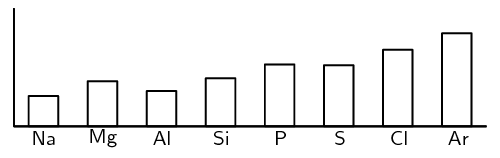Home Practice
For learners and parents For teachers and schools
Textbooks
Full catalogue
Pricing SupportLog in

We think you are located in United States. Is this correct?

# End of chapter exercises

## Periodic table

Textbook Exercise 5.3

For the following questions state whether they are true or false. If they are false, correct the statement

1. The group 1 elements are sometimes known as the alkali earth metals.

2. The group 8 elements are known as the noble gases.

3. Group 7 elements are very unreactive.

4. The transition elements are found between groups 3 and 4.

Solution not yet available.

Give one word or term for each of the following:

1. The energy that is needed to remove one electron from an atom

2. A horizontal row on the periodic table

3. A very reactive group of elements that is missing just one electron from their outer shells.

Solution not yet available.

Given $$_{35}^{80}\text{Br}$$ and $$_{17}^{35}\text{Cl}$$. Compare these elements in terms of the following properties. Explain the differences in each case.

2. Electronegativity

3. First ionisation energy

4. Boiling point

Solution not yet available.

Given the following table:

 Element $$\text{Na}$$ $$\text{Mg}$$ $$\text{Al}$$ $$\text{Si}$$ $$\text{P}$$ $$\text{S}$$ $$\text{Cl}$$ $$\text{Ar}$$ Atomic number 11 12 13 14 15 16 17 18 Density ($$\text{g·cm^{-3}}$$) $$\text{0,97}$$ $$\text{1,74}$$ $$\text{2,70}$$ $$\text{2,33}$$ $$\text{1,82}$$ $$\text{2,08}$$ $$\text{3,17}$$ $$\text{1,78}$$ Melting point (℃) $$\text{370,9}$$ $$\text{923,0}$$ $$\text{933,5}$$ $$\text{1 687}$$ $$\text{317,3}$$ $$\text{388,4}$$ $$\text{171,6}$$ $$\text{83,8}$$ Boiling point (℃) $$\text{1 156}$$ $$\text{1 363}$$ $$\text{2 792}$$ $$\text{3 538}$$ $$\text{550}$$ $$\text{717,8}$$ $$\text{239,1}$$ $$\text{87,3}$$ Electronegativity $$\text{0,93}$$ $$\text{1,31}$$ $$\text{1,61}$$ $$\text{1,90}$$ $$\text{2,19}$$ $$\text{2,58}$$ $$\text{3,16}$$ -

Draw graphs to show the patterns in the following physical properties:

1. Density

2. Boiling point

3. Melting point

4. Electronegativity

Solution not yet available.

A graph showing the pattern in first ionisation energy for the elements in period 3 is shown below:1. Explain the pattern by referring to the electron configuration

2. Predict the pattern in the first ionisation energy for the elements in period 2.

3. Draw a rough graph to show the pattern predicted in the previous question.

Solution not yet available.